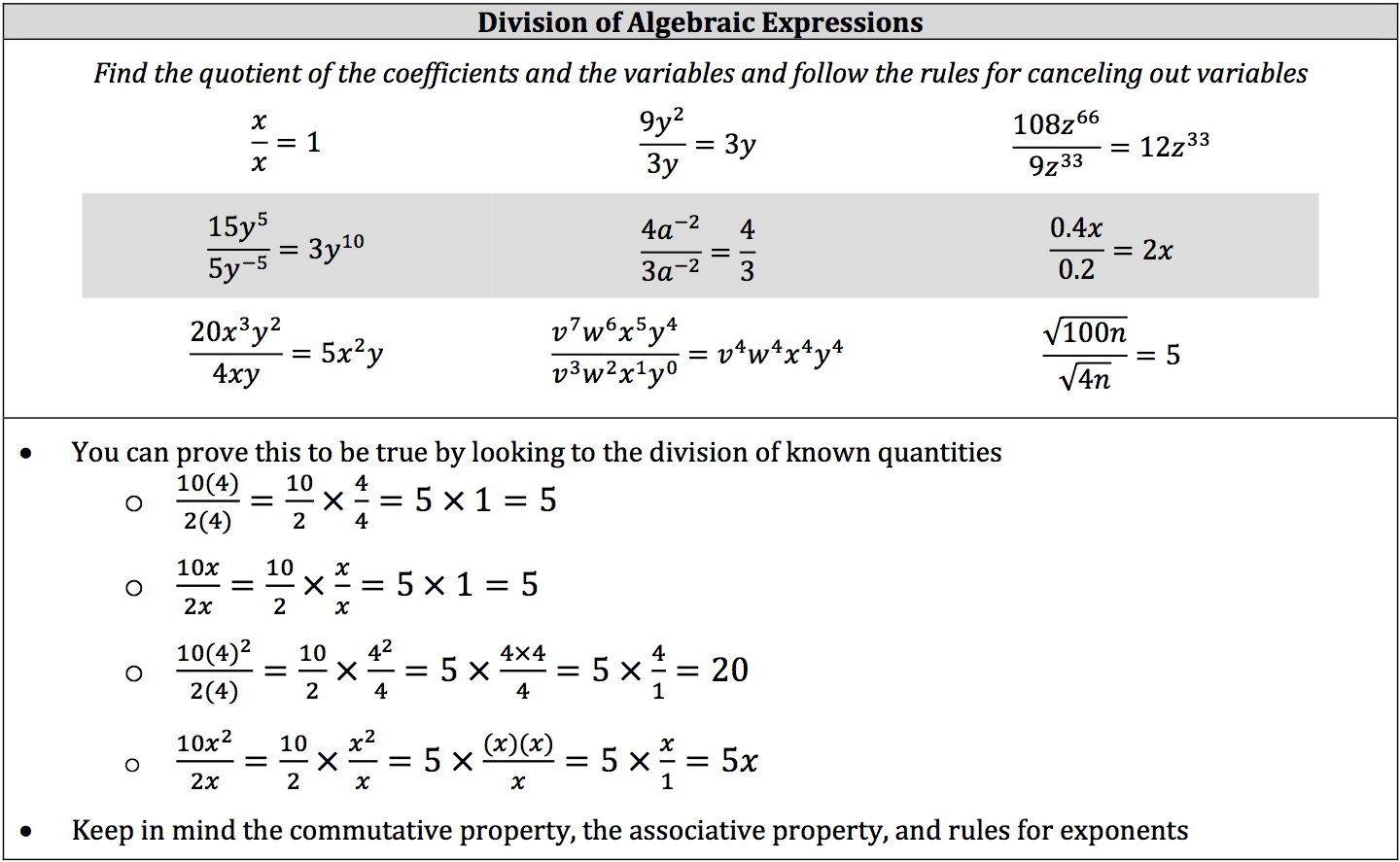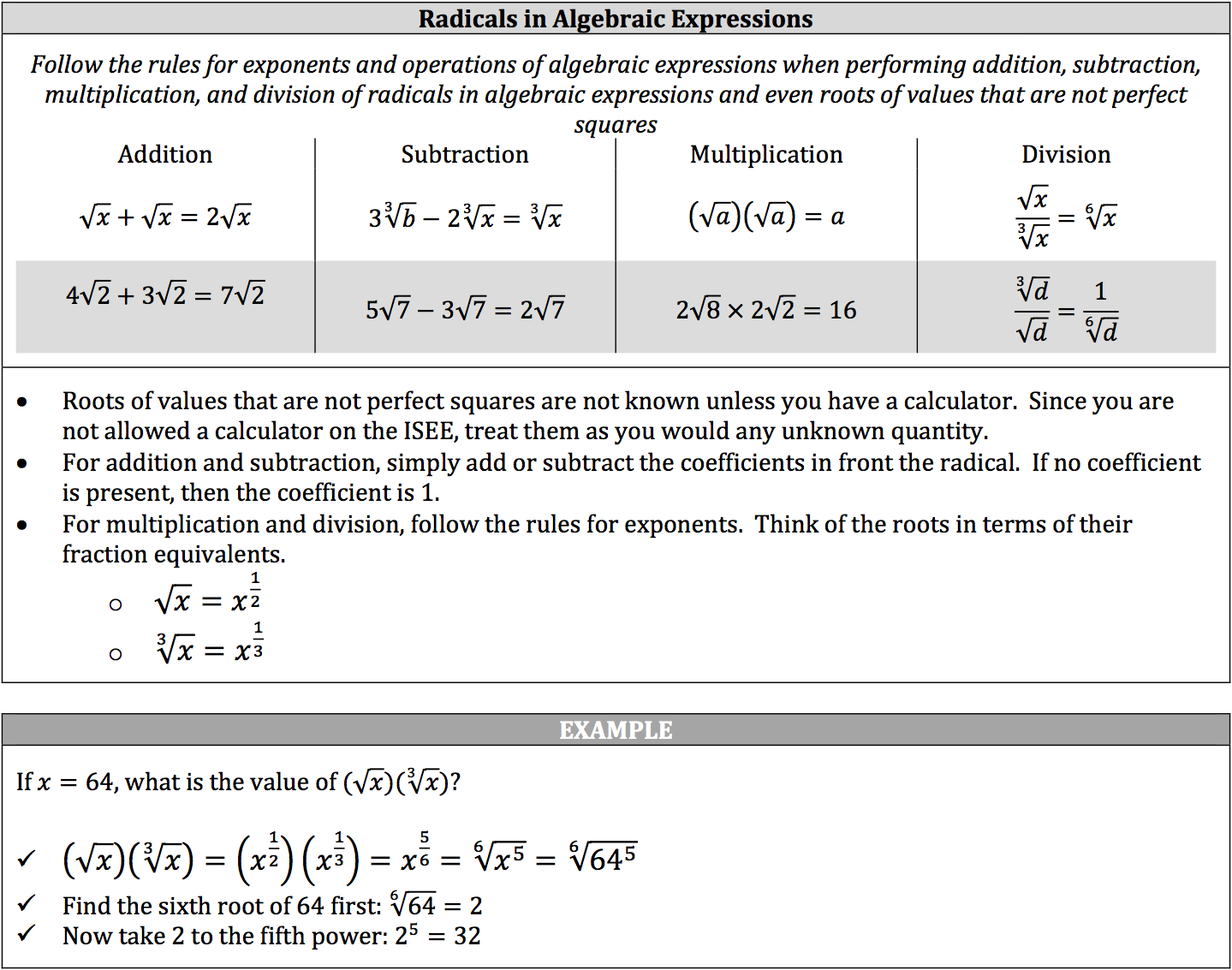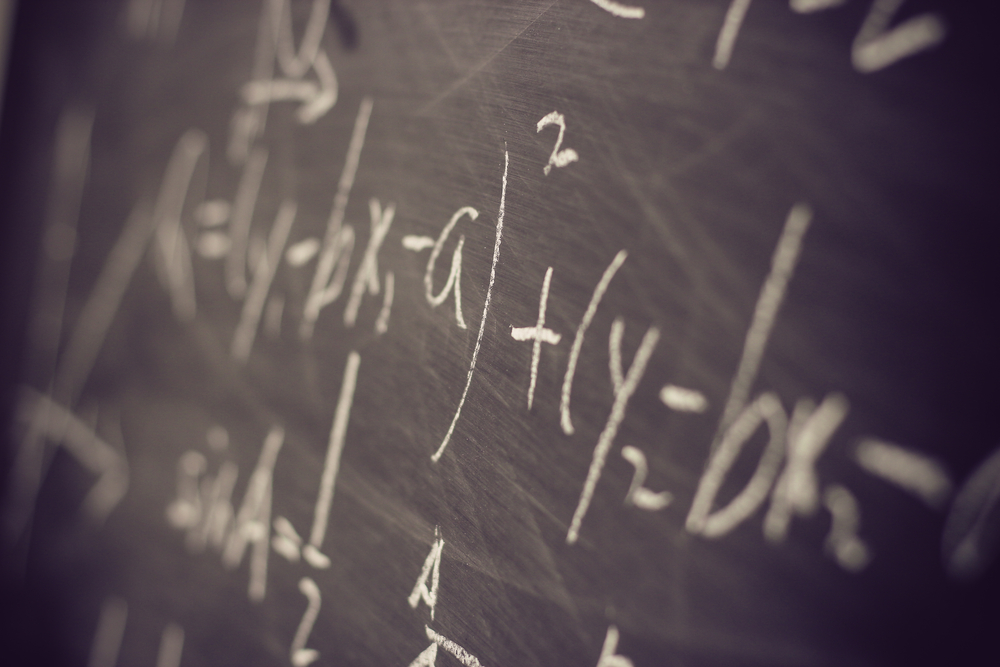In a nutshell, Algebra is arithmetic using known and unknown quantities. These unknown quantities are called variables and are represented as a letter (x) or a symbol. You have already evaluated some algebraic expressions by substituting certain values for some or all of the variables within the expression.

Now, let’s apply what we know about basic mathematical operations to algebraic expressions without substituting values for the variables.

Certain operations can only be performed when the variables are the same (“like terms”), and these operations generally involve the number attached to the variable, called the coefficient (ex. 9x; 9 is the coefficient). If no coefficient is present, then the coefficient is 1. The rules for addition, subtraction, multiplication, and division or known quantities are the same when applied to variables, including PEMDAS.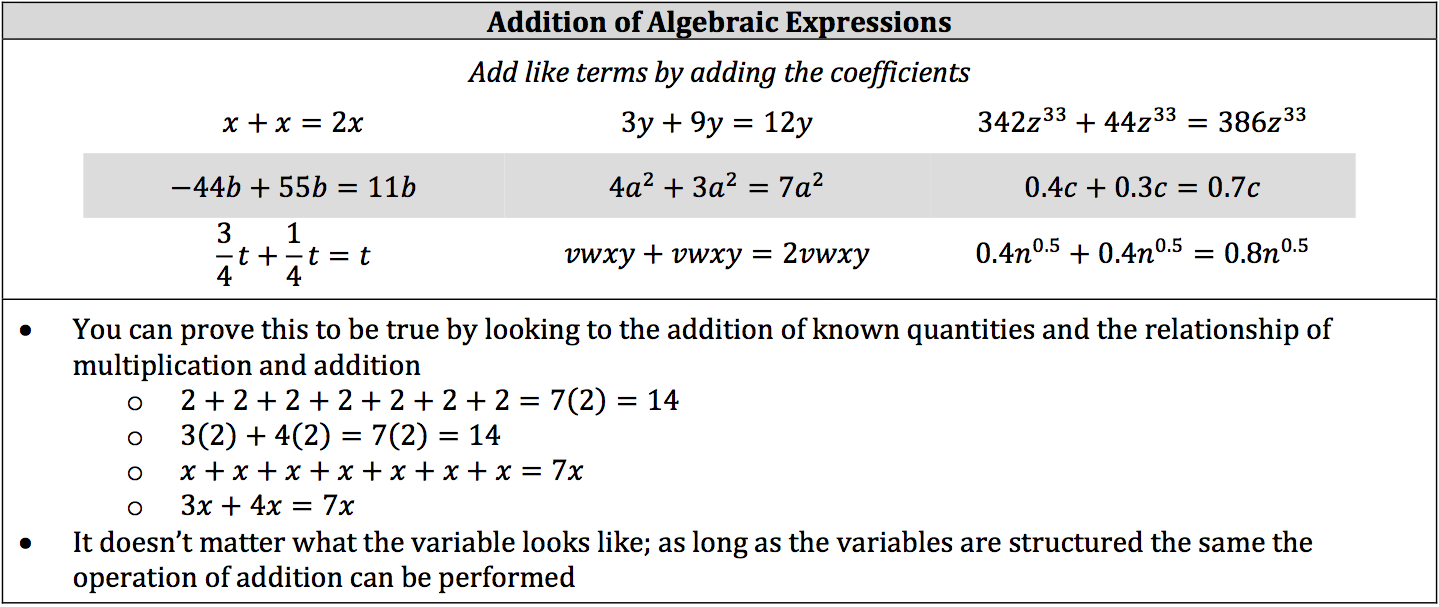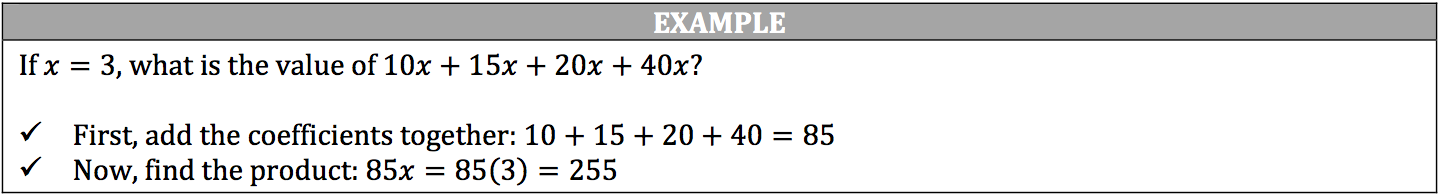### Subtraction of Algebraic Expressions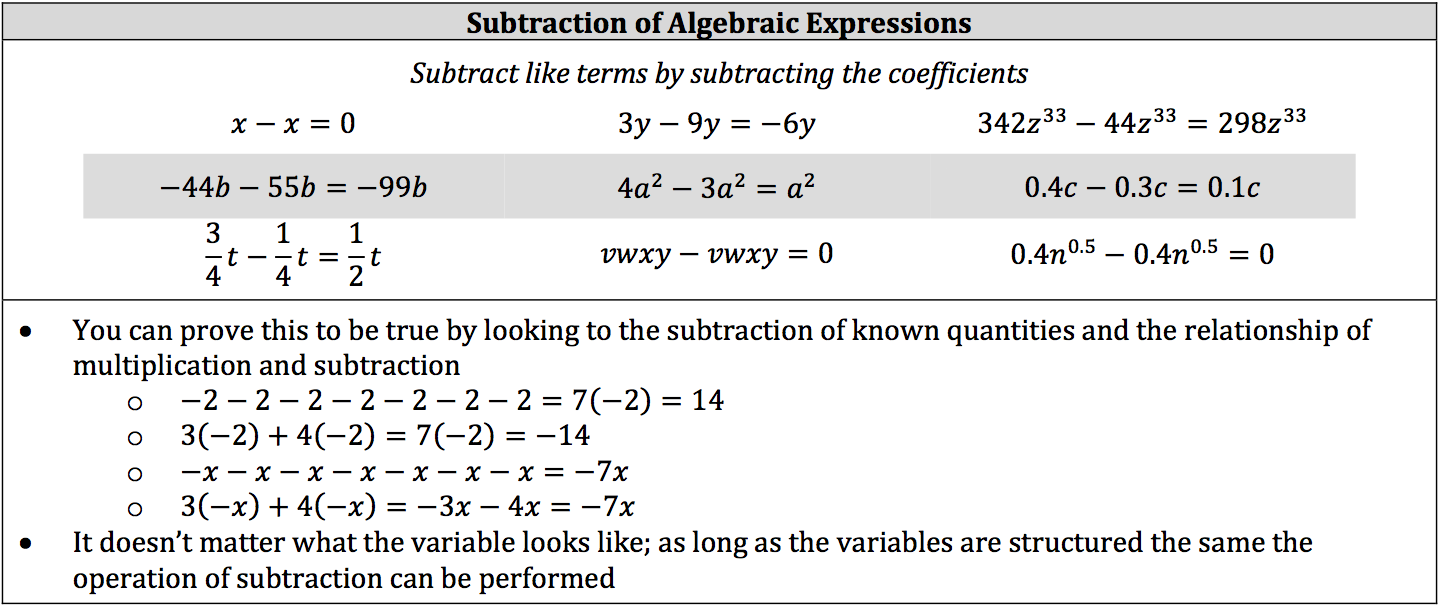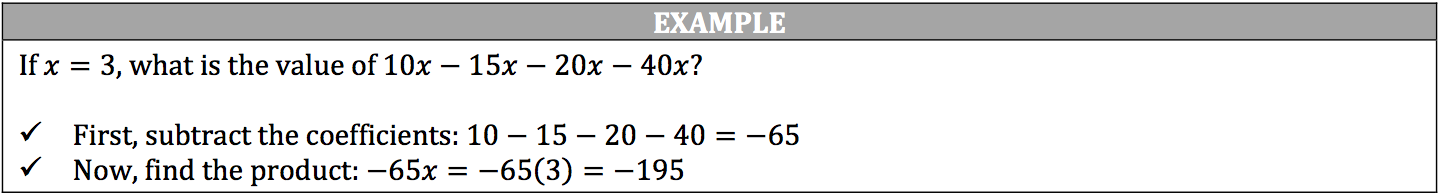### Multiplication of Algebraic Expressions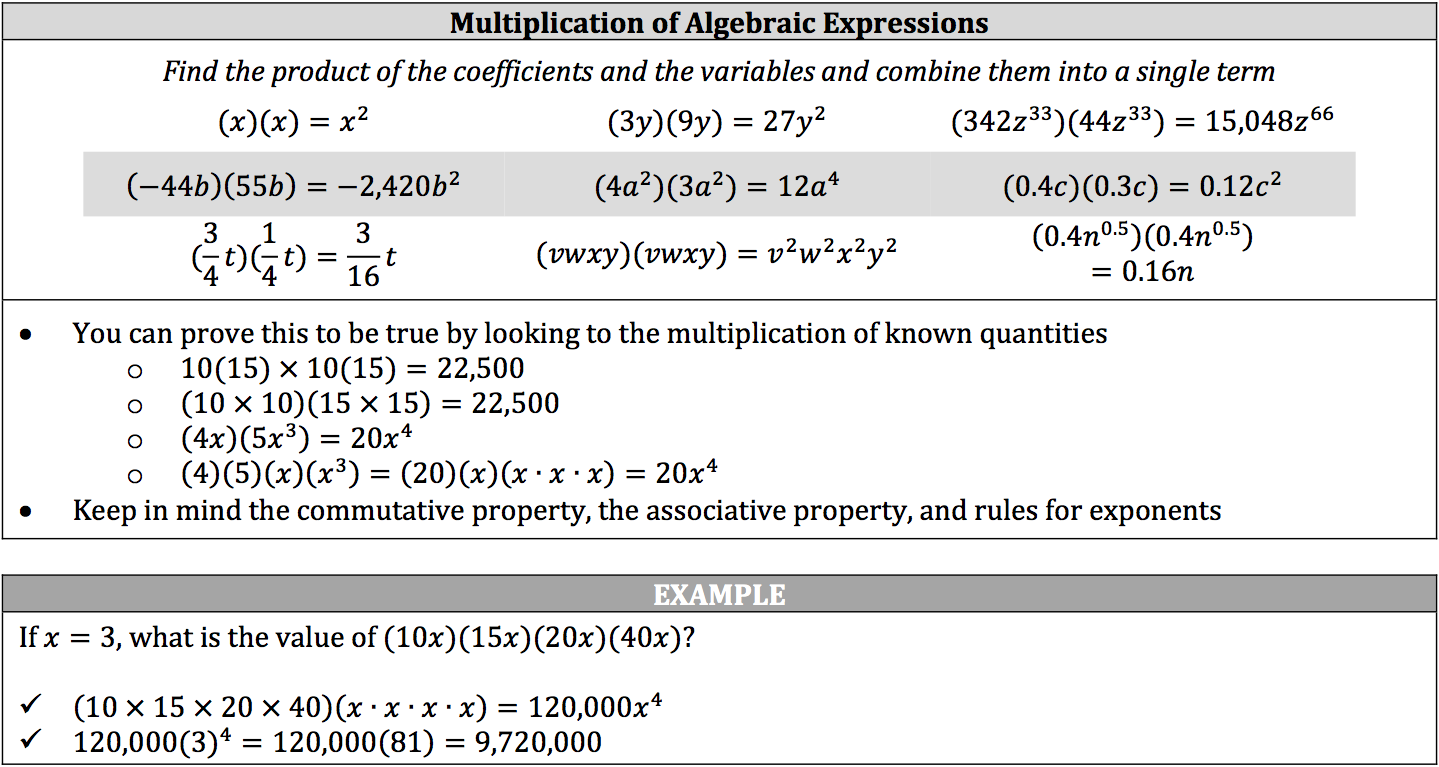### Division of Algebraic Expressions